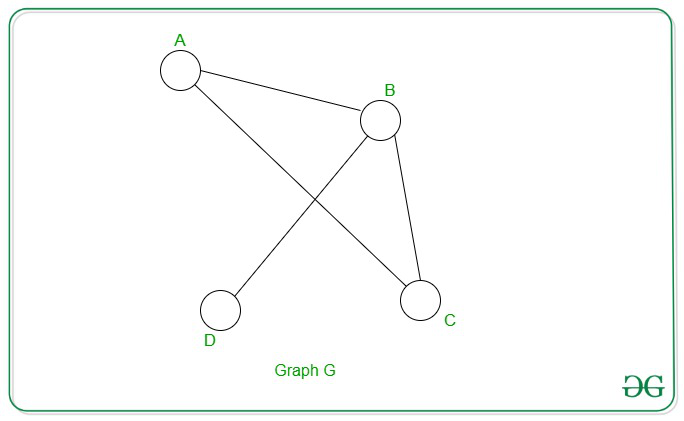# Optimized Longest Path is NP Complete

Optimized Longest Path Problem: The optimized longest path problem states that given a graph G, of a set of vertices V and edges E, the task is to prove that there is a path of length at least K between a set of nodes Vs and Ve.

Problem Statement: Given a graph G(V, E, K) and a set of nodes Vs and Ve with the sequence of nodes, of length ≥ K.

Explanation:
An instance of the problem is an input specified to the problem. An instance of the optimized-longest path problem is G(V, E, Vs, Ve, K). Since an NP-complete problem is a problem which is both in NP and NP-Hard, the proof for the statement that a problem is NP-Complete consists of two parts:

1. The problem itself is in NP class.
2. All other problems in NP class can be polynomial-time reducible to that.
(B is polynomial-time reducible to C is denoted as B≤PC)

If the 2nd condition is only satisfied then the problem is called NP-Hard.

But it is not possible to reduce every NP problem into another NP problem to show its NP-Completeness all the time. That is why if we want to show a problem is NP-Complete we just show that the problem is in NP and any NP-Complete problem is reducible to that then we are done, i.e. if B is NP-Complete and B ≤ PC For C in NP, then C is NP-Complete. Thus, we can verify that the Optimized Longest Path Problem is NP-Complete using the following two propositions:

• Optimized-longest Path Problem is in NP:
If any problem is in NP, given a ‘certificate’, which is a solution to the problem and an instance of the problem then it can be verified (check whether the solution given is correct or not) that the certificate in polynomial time. This can be done by a path P, consisting of a set of vertices < V1, V2, V3, ….Vn >. Verify if the path connects V1, and Vn completely and the length of the path is at most K.

• Optimized-longest Path Problem is NP-Hard:
In order to prove that the Longest Path is NP-Hard, deduce a reduction from a known NP-Hard to the problem. Carry out a reduction in which an undirected Hamiltonian Path problem can be reduced to the Longest Path problem. The undirected hamiltonian path uses the input a graph G(V1, Vn) where graph G has nodes V1 and Vn. Undirected Hamiltonian Path is an Undirected path along the graph starting at one vertex and ending at another traversing all nodes.

Now, let K be the numbers of nodes in G. Every instance of Undirected Hamiltonian Path can be converted to the Longest Path in the following way:
For input G(V1, Vn), output G(V1, Vn, k). This reduction takes polynomial time by simply counting the number of vertices in G. The reduction can be proved by the following two propositions:

1. Assume the original graph G(V, E) is provided with nodes V1 and Vn has an undirected Hamiltonian path, which traverses all the vertices, therefore G(V, E, K) is true because any two nodes in G will be connected by a path of length equal to its nodes i.e., K therefore Longest Path problem holds.
2. Let us assume the graph G'(V, E, Vs, Ve, K) has a Lpath of length K from Vs to Ve, which implies G’ contains a simple path of length K from Vs to Ve.
But, G contains K vertices, hence traverses all vertices starting at Vs and ending at Ve forming a hamiltonian path, G'(Vs, Ve).Let V1 ≡ B and Vn ≡ D
Now, G has an Undirected Hamiltonian Path ≡ BCAD of K = 4.
Therefore, G contains an optimized path of length = 4 between B and D.

Attention reader! Don’t stop learning now. Get hold of all the important DSA concepts with the DSA Self Paced Course at a student-friendly price and become industry ready.

My Personal Notes arrow_drop_upCheck out this Author's contributed articles.

If you like GeeksforGeeks and would like to contribute, you can also write an article using contribute.geeksforgeeks.org or mail your article to contribute@geeksforgeeks.org. See your article appearing on the GeeksforGeeks main page and help other Geeks.

Please Improve this article if you find anything incorrect by clicking on the "Improve Article" button below.

Article Tags :
Practice Tags :

Be the First to upvote.

Please write to us at contribute@geeksforgeeks.org to report any issue with the above content.